# Mathematics - Exponentiation (square, cube) - Power

Exponentiation is a binary operation involving two numbers:

1. the base (b) 1)
2. the exponent (n) (or index or power).

$$base^{exponent} = b^n = b_1 \times b_2 \times \dots \times b_n$$

## Example

$$2^3 = 2 \times 2 \times 2 = 8$$

## Text

In text notation or computer language, generally the exponentiation operator is noted ^

2^3 = 2 . 2 . 2 = 8


## Usage

### Permutation

The exponentiation is the formula to calculate the number of possible permutation (with repetition) in a set.

For instance, if you have a set of 3 elements {1,2,3}, if you can draw 2 elements, you have the following 9 possible selections (permutation):

• 1 1
• 1 2
• 1 3
• 2 1
• 2 2
• 2 3
• 3 1
• 3 2
• 3 3

that you can express with an exponentiation $$9 = 3^2 = b^n$$ because for each draw n, you have b choices. $$b_1 \times b_2 \times \dots \times b_n = b^n$$

### Base Calculation

You can even generalize the permutation calculation to base calculation (that's why b is called the base)

For instance, in a set of 10 digits { 0 , 1 , 2 , 3 , 4 , 5 , 6 , 7 , 8 , 9 }, if you can draw 2 numbers, you will have 100 possibilities (permutation). $$b^n = 10^2 = 100$$

Note that:

• a set of 10 digits in mathematics is also known as the base 10 used in the decimal system
• 100 is the maximal number that you can get with the digits (ie 99+0)

therefore the exponentiation gives you also the maximum number in any base for any length.

For instance, in base 2 (binary) with 8 digits (octet/byte), the maximum (decimal) number is $$b^n = 2^8 = 256$$ You can then translate any base into decimal. For instance, to translate the binary string 10101 into decimal: $$10101 = 1 \times \text{max of 4 digits} + 1 \times \text{max of 2 digits} + 1 \times \text{max of 0 digits} = 1 \times 2^4 + 1 \times 2^2 + 1 \times 2^0 = 16 + 4 + 1 = 21$$

## Pronunciation

The exponentiation $b^n$ can be read as:

• b raised to the n-th power,
• b raised to the power of n,
• b raised by the exponent of n,
• most briefly as b to the n.

Example: The Exponent raises the first number, the base, to the power of the second number, the exponent.

Some exponents have their own pronunciation.

### Squared

$b^2$ is usually read as b squared.

### Cubed

$b^3$ is usually read as b cubed.

Cubing a number is the same as raising it to the third power.

## Law

$$base^a * base^b = base^{a+b}$$

## When the exponent (n) is

### a positive integer

When the exponent (n) is a positive integer, exponentiation corresponds to repeated multiplication.

$$b^n = \underbrace{b \times \dots \times b}_{n}$$

It's a product of n factors, each of which is equal to b (the product itself can also be called power):

### even

The exponents of a product are all even, the product is a perfect square.

Example: $2^2*3^6 = (2*3^2)^2$

## Inverse

• With the below exponentiation

$$b^n = a$$

$$b = \sqrt[n]{a}$$

• While the logarithm permits to get the exponent,

$$n = log_b(a)$$

## Javascript

Array(8).fill().map((element,index) => console.log(2 pow ${index} is${Math.pow(2,index)}));


## Documentation / Reference

Recommended Pages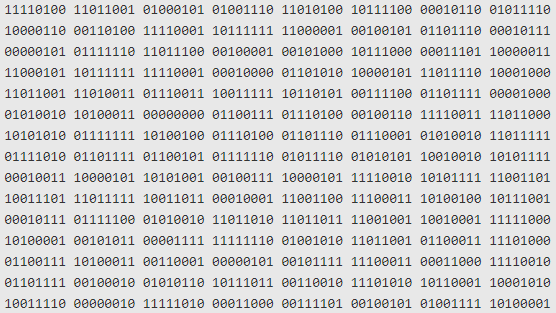Byte Array (multi-byte word)

A byte array is any array of byte. Library represents them generally as a multi-byte word with the possibility to change the endianess. See for instance, the Array Buffer in Javascript To a integer...Color - Luminance

Luminance is a photometric measure that is placed on a scale: that quantifies how a color is dark or light that goes from: 0 for darkest black to 1 for lightest white The ratio of luminance...Function - Binary Function/Operation

A binary operation is an scalar operation with two arguments (arity of two) that produces one value. They are creating a binary relation. the addition operator, the multiplication operator ...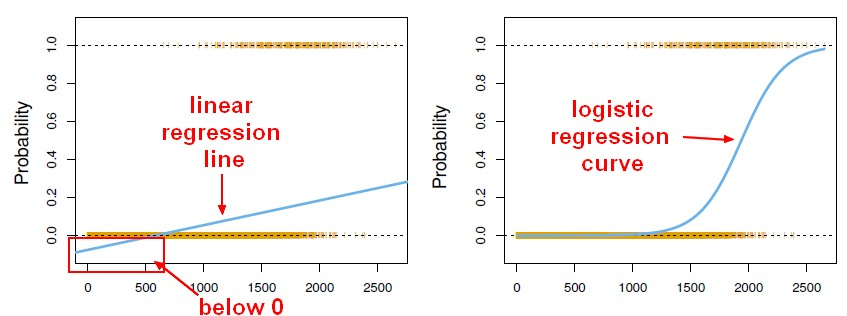Machine Learning - (Univariate|Simple) Logistic regression

A Simple Logistic regression is a Logistic regression with only one parameters. For the generalization (ie with more than one parameter), see Logistic regression comes from the fact that linear regression...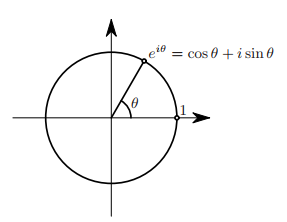Mathematics - Complex Exponential (Euler's formula)

Euler's formula provides a powerful connection between analysis and trigonometry, and provides an interpretation of the sine and cosine functions (the sinusoidal functions) as weighted sums of the exponential...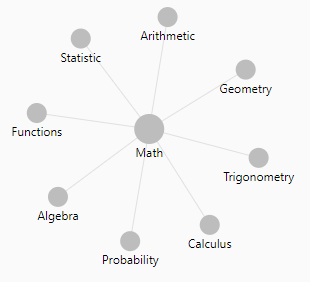Mathematics - Exponential (Euler's number)

is the scientific constant, the exponential. Euler's number The number e is an important mathematical constant that is the base of the natural logarithm. The number e can be defined to be: the unique...Mathematics - Inverse axiom

/ is inverse of - is inverse of + root is the inverse of exponentiation Functions f and g are functional inverses if: and are defined and are functions.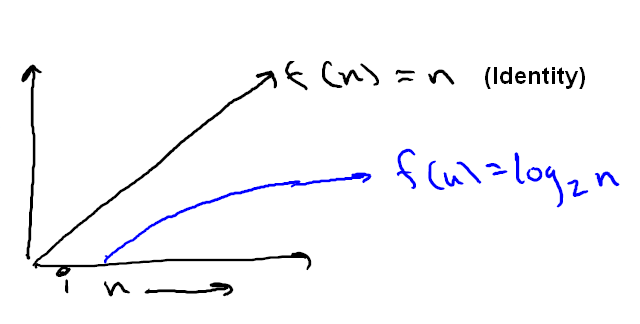Mathematics - Logarithm Function (log)

is the number (#) of times you divide n by b until you get down to 1. trees With the base 2 where is the # of times you divide n by 2 until you get down to 1. You keep repeating dividing by two...